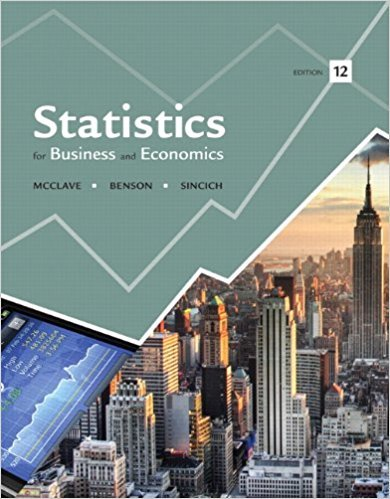×
Log in to StudySoup
Get Full Access to Statistics For Business And Economics - 12 Edition - Chapter 3 - Problem 1e
Join StudySoup for FREE
Get Full Access to Statistics For Business And Economics - 12 Edition - Chapter 3 - Problem 1e

Already have an account? Login here
×
Reset your password

# An experiment results in one of the following sampleISBN: 9780321826237 51

## Solution for problem 1E Chapter 3

Statistics for Business and Economics | 12th Edition

• Textbook Solutions
• 2901 Step-by-step solutions solved by professors and subject experts
• Get 24/7 help from StudySoup virtual teaching assistantsStatistics for Business and Economics | 12th Edition

4 5 1 335 Reviews
13
4
Problem 1E

Problem 1E

An experiment results in one of the following sample points: E1, E2, E3, E4, or E5.

a. Find P(E3) if P(E1) = .1, P(E2) = .2, P(E4) = .1, and P(E5) = .1.

b. Find P(E3) if P(E1) = P(E3), P(E2) = .1, P(E4) = .2, and P(E5) = .1.

c. Find P(E3) if P(E1) = P(E2) = P(E4) = P(E5) = .1.

Step-by-Step Solution:

Solution

Step 1 of 3

a) We have to findGivenWe know that the total probability is one++++=1

0.1+0.2++0.1+0.1=1

0.5+=1=1-0.5

=0.5

HenceStep 2 of 3

Step 3 of 3

##### ISBN: 9780321826237

Unlock Textbook Solution

Enter your email below to unlock your verified solution to:

An experiment results in one of the following sample